# A/B testing and Pearson’s chi-squared test of independence

A good friend of mine asked me recently about how to do A/B testing. As he explained, A/B testing refers to the process in which when someone visits a website, the site sends them to one of two (or possibly more) different ‘landing’ or home pages, and which one they are sent to is chosen at random. The purpose is to determine which page version generates a superior outcome, e.g. which page generates more advertising revenue, or which which page leads a greater proportion of visitors to continue visiting the site.

A/B testing is essentially a simple randomized trial – visitors to the webpage are randomized to either see landing page A or landing page B. Randomized trials are (usually) considered the gold standard study design for evaluating the efficacy of new medical treatments, but they are also used much more widely in experimental research. The key idea is that because you randomize which landing page (or treatment in the case of a randomized clinical trial) someone goes to, after a large number of visitors, the groups of people who visited the two pages are completely comparable in respect of all characteristics (e.g. age, gender, location, and anything else you can think of!). Because the two groups are comparable, we can compare the outcomes (e.g. amount of advertising revenue) between the two groups to obtain an unbiased, and fair, assessment of the relative effectiveness (in terms of our defined outcome) of the two designs.

Suppose for the moment that we’ve had two visitors to our site, and one visitor has been randomized to page A, and the other visitor to page B (note that it is entirely possible, with simple randomization, that both visitors could have both been sent to page A). Suppose next that the visitor to page A generated revenue, but the visitor to page B generated no revenue. Should we conclude that page A is superior to page B, in terms of revenue generation? Of course not. Because we have only sampled two visitors, it is entirely possible that the visitor to page A would have generated revenue even if they had been sent to page B, perhaps because they are very interested in the site’s content, whereas perhaps the visitor to page B was not particularly interested in the site content, and was never going to generate revenue.

We can overcome this problem by running the A/B testing for a sufficiently large number of visitors, such that the probability that the scenario described in the previous paragraph occurs is sufficiently small. Let’s suppose that we have obtained data from n visitors,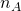of which have been (randomly) sent to page A, and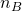of which have been sent to page B. Further, let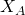and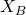denote the number of visitors for whom we obtained a ‘successful’ outcome in the two groups. The proportion of successes in the two groups is then given by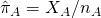and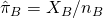respectively. The estimated difference in success rates is then give by the difference in proportions: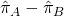To assess whether we have statistical evidence that the two pages’ success rates truely differ, we can perform a hypothesis test. The null hypothesis that we want to test is that the two pages’ true success rates are equal, i.e.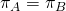, whereas the alternative is that they differ (one is higher than the other),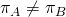. Or put another way, the null hypothesis says that the factors ‘page type’ and ‘outcome’ are statistically independent of each other. In words, this means knowing which page someone is sent to tells you nothing about the chance that they will have a successful outcome.

Pearson’s chi-squared test of independence
Assuming the samples aren’t too small, and the success rates too close to zero or one, we can use Pearson’s chi squared test (see later in the post for when this doesn’t hold). The basic idea of a hypothesis test is to define some function of the data, known as a test statistic, for which large values indicate the data are inconsistent with the null hypothesis. If we can derive the distribution of this test statistic (in repeated sampling) under the null hypothesis, the p-value is calculated as the probability (under the null) that the test statistic takes a value equal to, or more extreme (less consistent with the null) than the value observed.

In the case of Pearson’s chi squared test for the so called 2×2 table, the test statistic is the difference in the observed proportions, standardized by an estimate of the standard error of this quantity (calculated assuming the null hypothesis):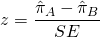where SE denotes the estimated standard error of the difference in proportions (under the null). To find this, we need to find the variance ofunder the null. This can be found as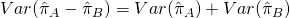since the two groups are statistically independent. The variance of a binomial proportion is given by (e.g. for group A)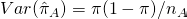where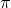denotes the true (assumed common) probability of success. Under the null, both groups provide an estimate of. This estimate is equal to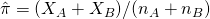Putting these together, we have that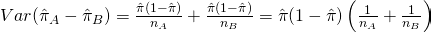The SE is then just the square root of this variance. So, our z-statistic is given by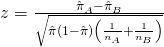We then compare our calculated z-statistic to the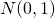distribution. Specifically, we find the probability that a standard normal variable is larger in absolute magnitude than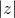(wheredenotes the absolute value of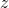). A simple rule is that if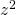is larger than 3.84 (the 95% centile of the chi-squared distribution on one degree of freedom), then the test is statistically significant at the 5% level. The 5% level is the usual (arbitrary!) significance level that is used. If your z-statistic is significant at the 5% level, we have reasonable evidence against the null hypothesis that the two pages are equally effective. Or put another way, we have reasonable evidence that the two pages are not equally effective.

Pearson’s chi-squared test of independence in R
If you want to calculate the actual p-value, you can do this using the following R code:

2*pnorm(-abs(z))

where z denotes your calculated z-statistic.

Of course, R provides a facility for doing all of this hard work for you! First, we need the data in a 2×2 matrix. Let’s say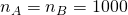,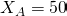, and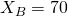. Then we can define

x <- matrix(c(50, 70, 950, 930), nrow=2)

Then we pass x to the chisq.test function:

chisq.test(x)

Pearson's Chi-squared test with Yates' continuity correction

data: x
X-squared = 3.2004, df = 1, p-value = 0.07362

The p-value is 0.07, so not quite significant at the 5% level - we do not have strong evidence against the null here.

Fisher's exact test
When one or more of the counts in the 2x2 table are small (i.e. <5) the asymptotic justification of Pearson's chi-squared test may not be appropriate. One alternative is Fisher's so called 'exact' test. The test tests the same null hypothesis of no association. It is calculated by conditioning on the marginal totals, and calculating the probability of observing the observed data or more extreme, under the null hypothesis. Unlike Pearson's test, it is valid even when one or more of the cells have small values. In R we can do this using fisher.test:

fisher.test(x)

Fisher's Exact Test for Count Data

data:  x
p-value = 0.07323
alternative hypothesis: true odds ratio is not equal to 1
95 percent confidence interval:
0.4709572 1.0322155
sample estimates:
odds ratio
0.6993767


Here the p-value (0.07323) is very similar to the one from Pearson’s chi-squared test, since none of the cell counts are small. We also see that the fisher.test function gives us the estimated odds ratio for the association between the two variables, and a 95% confidence interval for the odds ratio.

### 1 thought on “A/B testing and Pearson’s chi-squared test of independence”

1.Jonathan, good article.
But I have a question: you talk about chi-square, however what you actually describe is the procedure for an independent samples z-test, including the2*pnorm(-abs(z)).
I know that the z^2 equals the chi-square score, from this point of view, the two tests are identical. And since the z-test is so simple, why would anyone do a chi-square test for AB testing?